# The Pi Molecular Orbitals of Benzene

Last updated: May 4th, 2020 |

The Pi Molecular Orbitals of Benzene

Today, let’s go through how to draw out the molecular orbitals of benzene. We’ll compare them with the molecular orbitals for (linear) hexatriene. The big takeaway is that from contrasting the molecular orbitals of these two 6-electron pi systems, we will unlock the deep, mysterious riddle of why benzene is so unusually stable.

Quick spoiler. Here’s of what the molecular orbitals of benzene look like.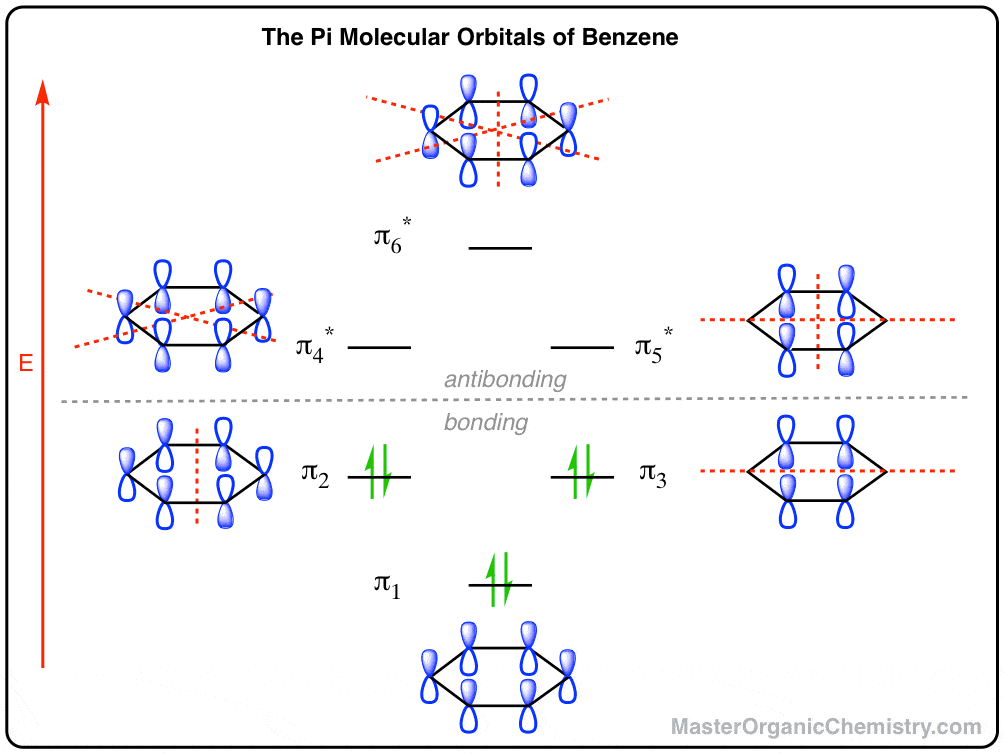The rest of this post will describe how we came up with this drawing and what it means.

## 1. Quickie Review: How To Draw Pi Molecular Orbitals For A Given Pi System

Previously we’ve looked at the molecular orbitals of the allyl system, and of butadiene. We learned some key lessons for drawing out the molecular orbitals of (linear) pi systems that I will quickly rehash here.

Think of drawing out the pi orbitals as a bit like constructing an apartment building, albeit with some strange municipal building codes and quirky tenant behaviour.

• The number of pi molecular orbitals in the pi-system equals the number of contributing atomic p orbitals.  For butadiene (n=4) we saw that the energy levels of the pi system stacked like a four-story apartment building.  Both hexatriene and benzene have six contributing p-orbitals  (n = 6), so we should expect six pi orbitals for each.
• The number of nodes increases with each successive energy level. A “node” is where there is a change in phase between adjacent p-orbitals (i.e. where they can’t constructively overlap). The lowest energy level (the “ground floor”, if you will) has all the p-orbitals aligned the same way, and therefore has zero nodes between the p orbitals (not counting the node inherent to the p-orbitals that lies in the plane of the molecule).  This provides the greatest possible delocalization of the electrons, and hence is the lowest in energy.  Here’s what the “ground floor” looks like for butadiene:The highest energy level (the “penthouse” of our building) has (n–1) nodes. We saw that for butadiene (n=4) the highest energy level has three nodes between the orbitals (marked here with red lines).The Ground Floor and the Penthouse are the easiest levels to draw, because they follow from simple rules: align all phases, or alternate all phases.

The tricky part is drawing the orbitals in the intermediate energy levels, due to the quantum-mechanical municipal bylaw that I call the Balanced Node Rule.  Because (math), nodes can’t just be placed anywhere; they’re always arranged symmetrically with the respect to the centre of the orbital.

• A single node must cut through the centre of the molecular orbital.
• Two nodes must be placed an equal distance from the centre (i.e. such that they are balanced with respect to the centre)
• Each successive energy level adds an extra node. Note that an orbital with an odd number of nodes will always have one node in the centre.

The final task in drawing the molecular orbitals is to fill up our building with tenants (electrons) using the familiar Aufbau principle: starting with the ground floor, each unit (orbital) fills up one electron at a time, to a maximum occupancy of two.1

Now let’s apply this framework to hexatriene and benzene.

## 2. Building The Pi Molecular Orbital Diagram For Benzene: Hexatriene and Benzene Each Have Six Pi Molecular Orbitals

Hopefully straightforward!  Six p orbitals in the pi systems of benzene and hexatriene will produce six pi molecular orbitals.

The levels in hexatriene stack like a six-story building. Benzene has a different arrangement, for reasons we’ll quickly see below.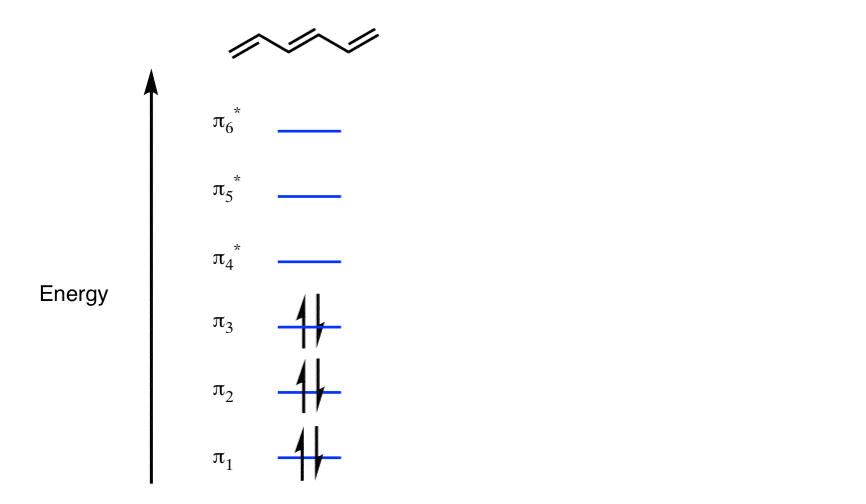Note that the bottom three orbitals are all bonding orbitals and the top three orbitals are antibonding. Only the bottom three “floors” are occupied in neutral hexatriene.   (If every floor in this building were completely filled with tenants (electrons) the building would quickly self-destruct. Talk about a fucked-up building)

## 3. The Lowest-Energy Molecular Orbitals Of Hexatriene And Benzene Have Zero Nodes

Following the pattern described above for butadiene, we draw the “ground floor” first, with the phases of all p-orbitals aligned the same way. It doesn’t matter if you draw the “shaded” or “white” lobes up or down, so long as they are all drawn the same way.

Here’s what they look like for hexatriene and benzene: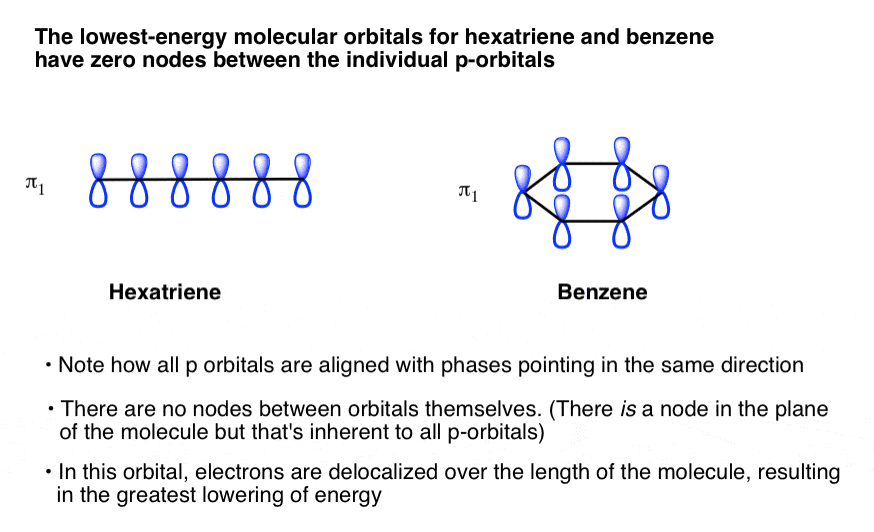## 4.  The “Penthouse” Of The M.O. Diagram  (Highest Energy Level) Has The Maximum Number Of Nodes

The Highest Energy molecular orbitals  have p orbitals with completely alternating phases.

Like the ground floor, the highest-energy molecular orbital (the “penthouse”)  of a pi system is also straightforward to draw.

Draw all the p orbitals with alternating phases. No two adjacent p orbitals should have lobes with the same phase.

For linear systems, we’ve seen that this gives the highest energy level  (n–1) nodes. For hexatriene (n=6) that means that the highest energy level will have 5 nodes.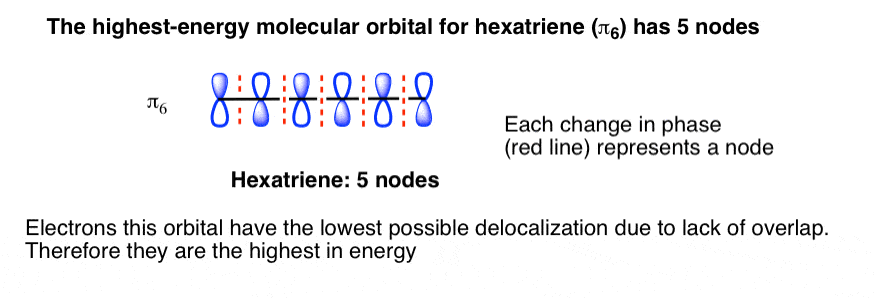What about benzene? This is where things get interesting.

## 5. Benzene Has Nodal Planes. The Maximum Energy Level Has 3 Nodal Planes

In the case of cyclic systems, the (n–1) rule fails. Drawing a molecular orbital of benzene with 5 nodes is like solving 5 faces of a Rubik’s cube: impossible. A meelion dollars if you can prove me wrong: try it!

We can, however, easily draw an orbital where all the phases alternate. In this case, though, we can count six places where the phases change. Instead of looking at these as individual nodes, it’s perhaps more helpful to think of these as three nodal planes, which cut through the molecule at various points.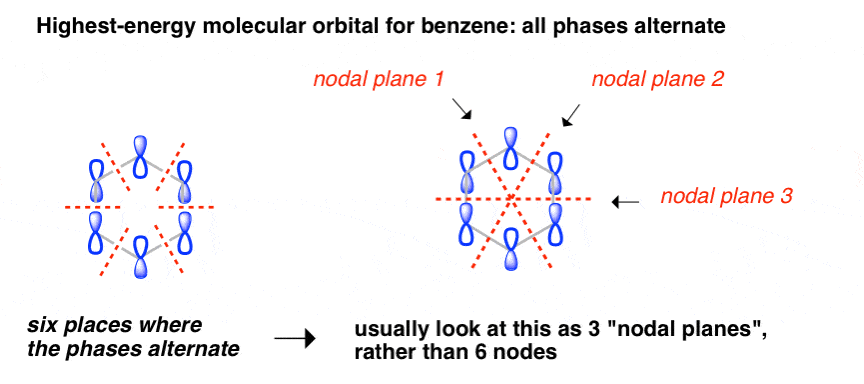This orbital has zero overlap between adjacent p orbitals and therefore electrons in this orbital have the minimum possible delocalization. They are therefore the highest energy.

## 6. Where Do We Place The Nodes In The Intermediate Energy Levels Of Benzene?

As we said above, the tricky thing in building pi molecular orbitals is knowing where to put the nodes in the intermediate levels.

For hexatriene, the second floor (one node) is fairly straightfoward: we put the node in the centre, like this:It’s impossible to draw a cyclic pi system with one node, but we can draw a system with one nodal plane. Here, for instance, we’ve drawn a nodal plane that cuts through two of the single bonds:But wait! There’s actually a second way to do it. We can also draw a nodal plane through the atoms, like this.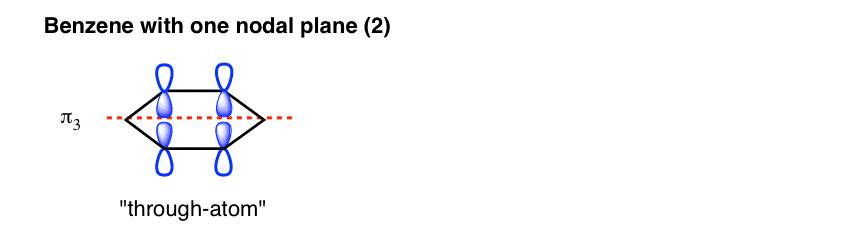These two molecular orbitals (πand π) have the same number of nodal planes, and therefore have the same energy. The way we usually describe this in chemistry is by saying the orbitals are degenerate.

This is really the key difference in the molecular orbital picture of a cyclic system versus an acyclic system: two units can co-exist on the same floor. For benzene, that results in a lowering of energy.

## 7. Filling Out The Rest of The Picture For Benzene

Here’s the third, fourth, and fifth “floors” for the hexatriene pi system, which have two, three, and four nodes, respectively.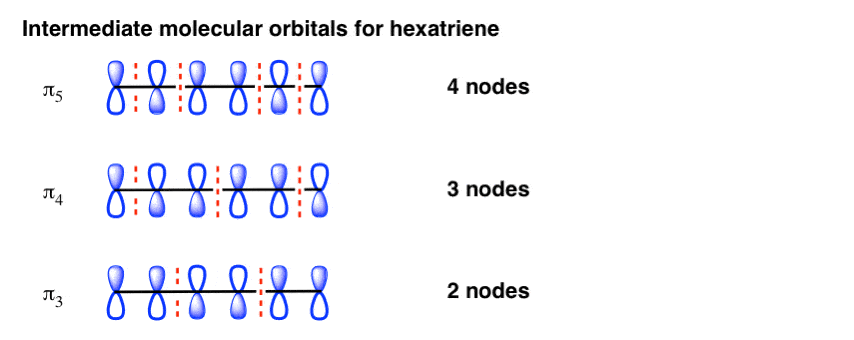For benzene, the next level up has two nodal planes. Again, there’s two ways to do it: cut through the bonds, or cut through the atoms. Again, these are of the same energy.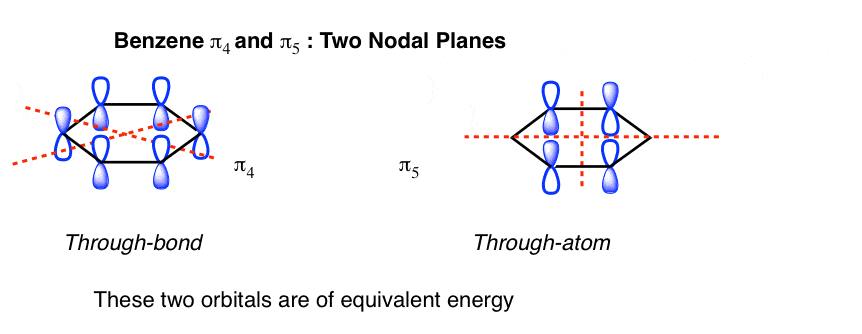## 8. The Benzene Molecular Orbital Diagram: Putting It All Together

The final step is to arrange all the pi orbitals together (by “floors”) and then to fill each level with electrons (“tenants”).

Here’s the complete molecular orbital picture for hexatriene: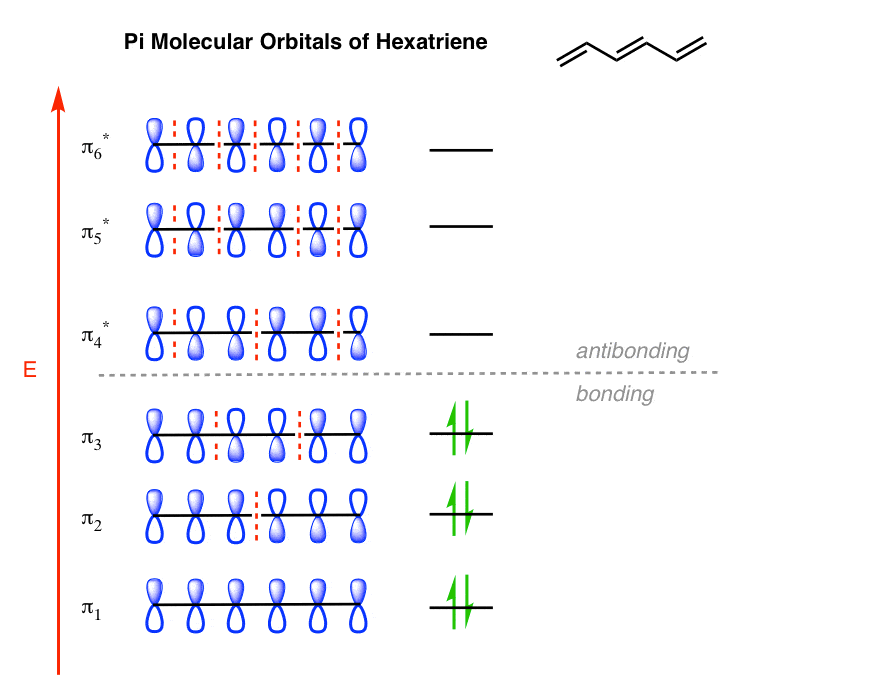The complete molecular orbital picture for benzene was drawn at the top of the post, but here it is again for your non-scrolling pleasure: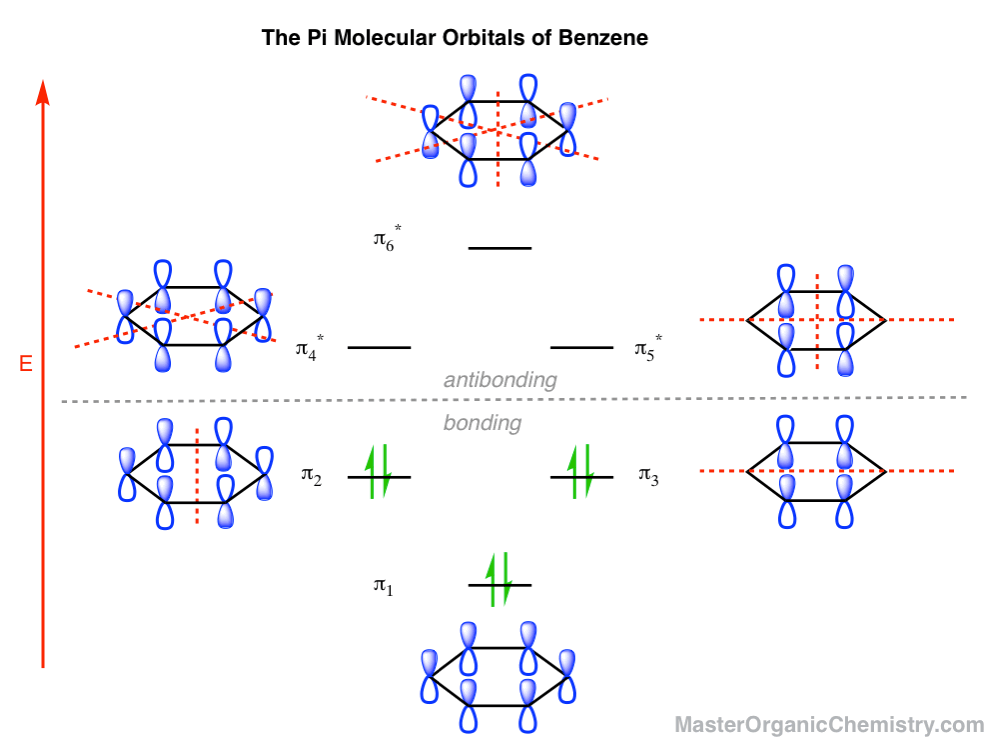See the key difference? Describing it as “a more efficient stacking of energy levels” isn’t far off.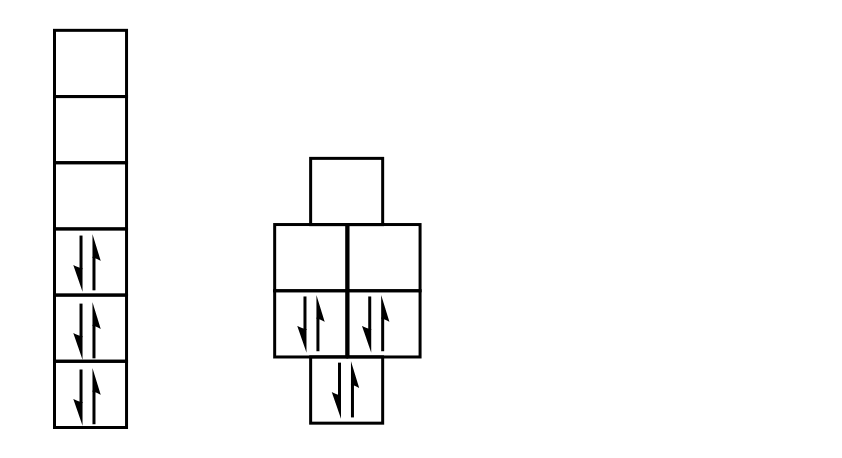## 9. Why Is Benzene More Stable Than Hexatriene?

A cyclic pi system allows for two ways to place a single nodal plane: through-bond, or through-atom. In benzene, this means that the second and third “floors” each have two units with the same energy – they are “degenerate”.

To follow our somewhat crude analogy, both buildings have six “tenants”, but in benzene, those tenants aren’t as high off the ground – and thus have less potential energy.

In chemistry terms,  the highest occupied molecular orbitals (HOMO) of benzene are lower in energy than the highest occupied molecular orbital (HOMO) of hexatriene. And for our purposes, that lower energy of the pi-electrons translates into lower reactivity. [Note 3]

It’s an imperfect analogy, but for our purposes, it will do.

In the next post, let’s see if we can examine the molecular orbitals for anti-aromatic cyclobutadiene and similarly gain insights into its unusual instability.

## Comment section

### 8 thoughts on “The Pi Molecular Orbitals of Benzene”

1.mamid says:

The leftmost orbital in the pi-6 molecular orbital of benzene should be turned upside down, shouldn’t it?

1.James says:

Fixed. Thank you so much, again.

2.Hayder says:

Thank you very much

1.James Ashenhurst says:

3.Rangan says:

Can we say this degeneracy in benzene is reflected in Hückel’s (4n+2) rule of aromaticity ?

1.James Ashenhurst says:

No, (4n+2) is just an algebraic expression of the series 2,6,10,14…. It has no physical meaning.

4.S. Lin says:

Could you please explain why cyclopentadienyl cation is anti-aromatic? I know it has 4n pi electrons but the MO diagram indicates that it does not occupy any antibonding MO, it just does not fill bonding MO, what makes it very unstable? Is it because the electrons are not paired up in pi 2 and pi 3 MOs? Thank you very much.

1.James Ashenhurst says:

To build the MO diagram, I would use a Frost Circle of an pentagon with the apex pointing down. You’ll have the first two pi electrons go in the bottom most level (the apex), but the next two energy levels are degenerate and are half-filled. This is the problem: the molecule has diradical character with two half-filled orbitals of equal energy, a very unstable situation.

This site uses Akismet to reduce spam. Learn how your comment data is processed.